SEARCH HOMEMath Central Quandaries & QueriesQuestion from Stephanie, a student: I'm trying to make a cone out of a flat sheet of metal for a "claw setting" for a gemstone. The cone must be 8mm wide at the top and 11mm long tapering to a point. But because the prongs must be cut out of the top the cone should not start to taper for a length of 3mm from that top 8mm. The 3mm prong is then bent over the 8mm stone. That probably doesn't make enough sense. But I don't know how to explain it. If it helps a claw setting is the very common prong setting for engagement rings or earrings. Please help as soon as possible as this is a commissioned piece for someone and I'm running out of time. I don't remember any math really from high school so please make the instructions really easy to follow. Thank You!!Hi Stephanie,

First consider the cone without the claws so the cone is 8 mm long. We will worry about the claws later.

I am not sure what measurement you are calling the length. Is it h or s? h is called the height and s the slant height.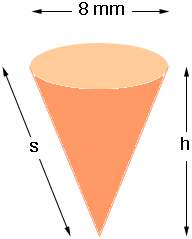I am going to assume that the h= 8 mm.

If you slice up the side of the cone and roll it out flat you have a sector of a circle of radius s mm.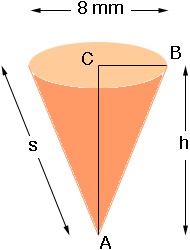Triangle ABC is a right triangle and Pythagoras' theorem then tell us that

|AB|2 = |BC|2 + |CA|2

thus

s2 = 42 + 82 or s = 4 √5 mm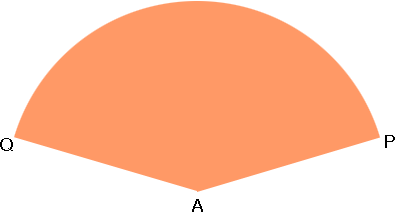Thus the distance |AP| = 4 √5 mm and the arc from P to Q is the circumference of the circle at the top of the cone which is 2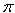r = 8mm.

We need the angle at A which is a fraction of 360 degrees. The length of the arc PQ is also a fraction of the circumference of the entire circle of radius 4 √5 mm. This circumference 2r = 8 √5mm. From the symmetry these fractions are the same which means

(angle at A)/360 = 8/(8 √5)

thus

the angle at A measures 360/√5 = 161 degrees.

Draw a circle of radius 4 √5 = 8.9 mm and mark off a sector with the central angle 161 degrees. Draw another circle of radius 8.9 + 3 = 11.9 mm with the same centre.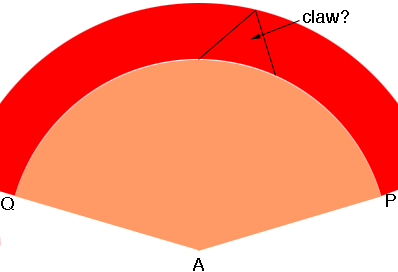Cut the claws from the band between the two circles and roll it up to form the cone and the claws will be 3 mm long.

PennyMath Central is supported by the University of Regina and The Pacific Institute for the Mathematical Sciences.